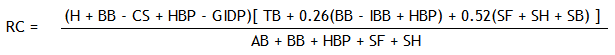# Runs Created Calculator & Formula

Runs Created Calculator
At-Bats   AB =
Bases on Ball   BB =
Hits   H   =
Doubles   2B =
Triples   3B =
Home Runs   HR =
Stolen Bases   SB =
Caught Stealing   CS =

In 1982, the baseball statistician Bill James developed the Runs Created sabermetric, abbreviated RC. Runs created is just what its name indicates, the number of runs that a player creates for his team, whether by direct or indirect action. It is a useful metric to compare with other batting metrics such as slugging percentage, on-base percentage, batting average, and extrapolated runs.

### Basic Formula

There are several formulas for computing RC depending on how many variables you want to consider. The simplest version of the formula is

RC = TB(H + BB)/(AB + BB),

where TB is total bases, BB is bases on balls (walks), H is hits, and AB is at-bats. This formula multiplies the total bases achieved by an on-base factor (H + BB) and then divides by an opportunity factor (AB + BB).

If TB is not given in the stats tables, it can be computed with the formula

TB = H + 2B + 2*3B + 3*HR

### Alternate Version: Stolen Bases

Another version of RC takes into account the number of stolen bases, which creates a more realistic metric of a players ability to create runs:

RC = (H + BB - CS)(TB + 0.55*SB)/(AB + BB).

In this formula, CS is caught stealing and SB is stolen bases. This is the RC formula programmed into the calculator above.

### Technical Refinements

James later created 14 more technical RC formulas that take into account all of the available offensive stats. These RC equations are known as Tech-1, Tech-2, etc. and each is applied to a particular period in American League and National League Baseball. Tech-1 can be applied to any year; its formula isHere, HBP is hits by pitch, GIDP is grounded into double play, IBB is intentional bases on balls, SF is sacrifice flies, and SH is sacrifice hits.

Example Calculation: A player's stats for one season are

1B = 55
2B = 20
3B = 5
HR = 40
(H = 120)
(TB = 270)
AB = 400
BB = 100
SB = 15
CS = 5

If we compute his runs created with the second formula, we have

RC = (120 + 100 - 5)(270 + 0.55*15)/(400 + 100)
= 215*278.25/500
= 120 (rounded to nearest whole number)